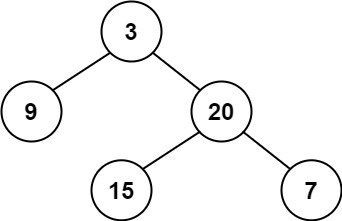## 110. Balanced Binary Tree

Given a binary tree, determine if it is height-balanced.

For this problem, a height-balanced binary tree is defined as:

a binary tree in which the left and right subtrees of every node differ in height by no more than 1.

Example 1:```Input: root = [3,9,20,null,null,15,7]
Output: true
```

Example 2:```Input: root = [1,2,2,3,3,null,null,4,4]
Output: false
```

Example 3:

```Input: root = []
Output: true
```

Constraints:

• The number of nodes in the tree is in the range `[0, 5000]`.
• `-104 <= Node.val <= 104`

## Rust Solution

``````struct Solution;
use rustgym_util::*;

trait Height {
fn height(&self) -> usize;
}

fn height(&self) -> usize {
match self {
None => 0,
Some(node) => {
let node = node.borrow();
usize::max(node.left.height(), node.right.height()) + 1
}
}
}
}

trait Balanced {
fn is_balanced(&self) -> bool;
}

fn is_balanced(&self) -> bool {
match self {
None => true,
Some(node) => {
let node = node.borrow();
let left = &node.left;
let right = &node.right;
let height_left = left.height();
let height_right = right.height();
if height_left == height_right
|| height_left == height_right + 1
|| height_left + 1 == height_right
{
left.is_balanced() && right.is_balanced()
} else {
false
}
}
}
}
}

impl Solution {
fn is_balanced(root: TreeLink) -> bool {
root.is_balanced()
}
}

#[test]
fn test() {
let a = tree!(3, tree!(9), tree!(20, tree!(15), tree!(7)));
assert_eq!(Solution::is_balanced(a), true);
let b = tree!(
1,
tree!(2, tree!(3, tree!(4), tree!(4)), tree!(3)),
tree!(2)
);
assert_eq!(Solution::is_balanced(b), false);
}
``````

Having problems with this solution? Click here to submit an issue on github.